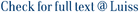Adopting a probabilistic approach we determine the optimal dividend payout policy of a firm whose surplus process follows a controlled arithmetic Brownian motion and whose cash-flows are discounted at a stochastic dynamic rate. Dividends can be paid to shareholders at unrestricted rates so that the problem is cast as one of singular stochastic control. The stochastic interest rate is modeled by a Cox–Ingersoll–Ross (CIR) process and the firm's objective is to maximize the total expected flow of discounted dividends until a possible insolvency time. We find an optimal dividend payout policy which is such that the surplus process is kept below an endogenously determined stochastic threshold expressed as a decreasing continuous function (Formula presented.) of the current interest rate value. We also prove that the value function of the singular control problem solves a variational inequality associated to a second-order, non-degenerate elliptic operator, with a gradient&nbsp;constraint.

Optimal dividend payout under stochastic discounting / Bandini, Elena; De Angelis, T.; Ferrari, G.; Gozzi, Fausto. - In: MATHEMATICAL FINANCE. - ISSN 0960-1627. - 32:2(2022), pp. 627-677. [10.1111/mafi.12339]

### Optimal dividend payout under stochastic discounting

#### Abstract

Adopting a probabilistic approach we determine the optimal dividend payout policy of a firm whose surplus process follows a controlled arithmetic Brownian motion and whose cash-flows are discounted at a stochastic dynamic rate. Dividends can be paid to shareholders at unrestricted rates so that the problem is cast as one of singular stochastic control. The stochastic interest rate is modeled by a Cox–Ingersoll–Ross (CIR) process and the firm's objective is to maximize the total expected flow of discounted dividends until a possible insolvency time. We find an optimal dividend payout policy which is such that the surplus process is kept below an endogenously determined stochastic threshold expressed as a decreasing continuous function (Formula presented.) of the current interest rate value. We also prove that the value function of the singular control problem solves a variational inequality associated to a second-order, non-degenerate elliptic operator, with a gradient constraint.
##### Scheda breve Scheda completa Scheda completa (DC)2022
Optimal dividend payout under stochastic discounting / Bandini, Elena; De Angelis, T.; Ferrari, G.; Gozzi, Fausto. - In: MATHEMATICAL FINANCE. - ISSN 0960-1627. - 32:2(2022), pp. 627-677. [10.1111/mafi.12339]
File in questo prodotto:
File
Mathematical Finance - 2022 - Bandini - Optimal dividend payout under stochastic discounting.pdf

Open Access

Descrizione: Articolo principale
Tipologia: Versione dell'editore
Licenza: Creative commons
Dimensione 1.32 MB
Utilizza questo identificativo per citare o creare un link a questo documento: `https://hdl.handle.net/11385/215243`
•3
•3